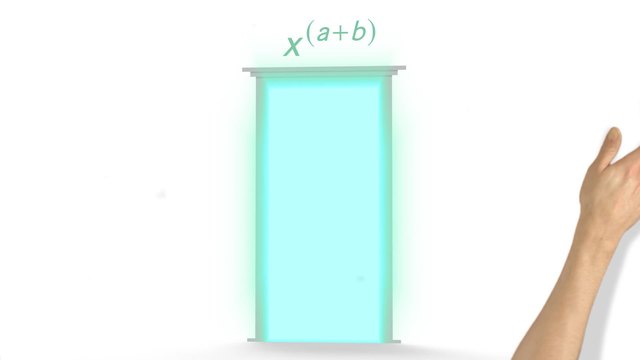# Proofs of Laws of ExponentsRate this video

Be the first to rate this video!

The authorChris S.

## Information about the videoProofs of Laws of Exponents

After this lesson you will be able to prove that a non-zero number raised to the zeroth power is equal to one.

The lesson begins by looking at the exponential pattern of two. It leads to using the law of exponents to define the zeroth power law. It concludes with applying the zeroth power law to all sorts of expressions.

Learn that a number raised to the zeroth power is equal to one by helping Vonn continue his studies in becoming a great philosopher!

This video includes key concepts, notation, and vocabulary such as: quotient of powers law (when dividing two powers with the same base, subtract the exponents); powers or power law (multiply the inner and outer exponents together); powers of products law (expand an expression by rewriting each terms with the outer exponent); and zeroth power law (any non-zero number raised to the zeroth power equals one).

Before watching this video, you should already be familiar with non-zero exponents.

After watching this video, you will be prepared to learn how to apply the laws of exponents to expressions with fractional and negative exponents.

Common Core Standard(s) in focus: 8.EE.A.1 A video intended for math students in the 8th grade Recommended for students who are 13-14 years old